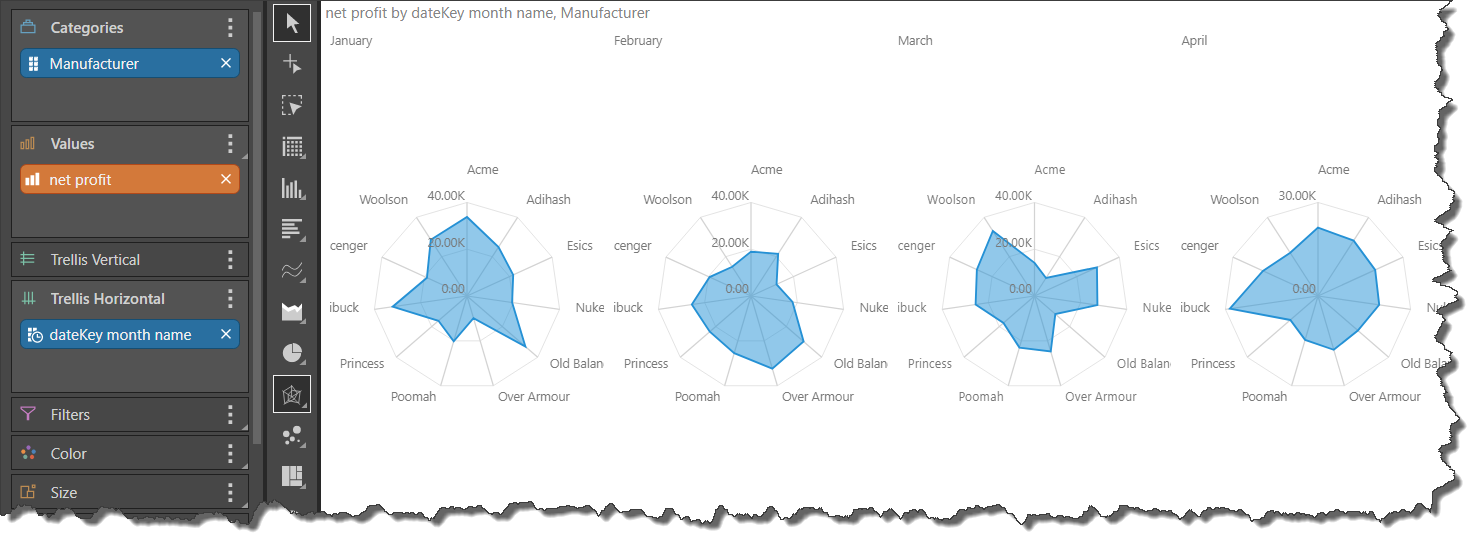Radar charts are used to plot one (or more) series of values across multiple quantitative variables. These quantitative variables are represented on axes extending from a central point. These axes are often referred to as spokes or radii. The angle, position, and length of these axes are uniform. The query must contain at least quantitative variables to produce enough axes in order to draw the chart.

The spread of values is marked on the upper middle axis, with concentric grid lines expanding from the central point outward. One or more series of values is overlaid on the chart, with the data points for each series plotted on each axis. Typically, the data points are joined together by straight lines, or shaded in to create polygon;. Pyramid offers additional variations on the standard radar chart.

Radar charts are typically used to see the spread of values in a series, or to see the variation between multiple series of values.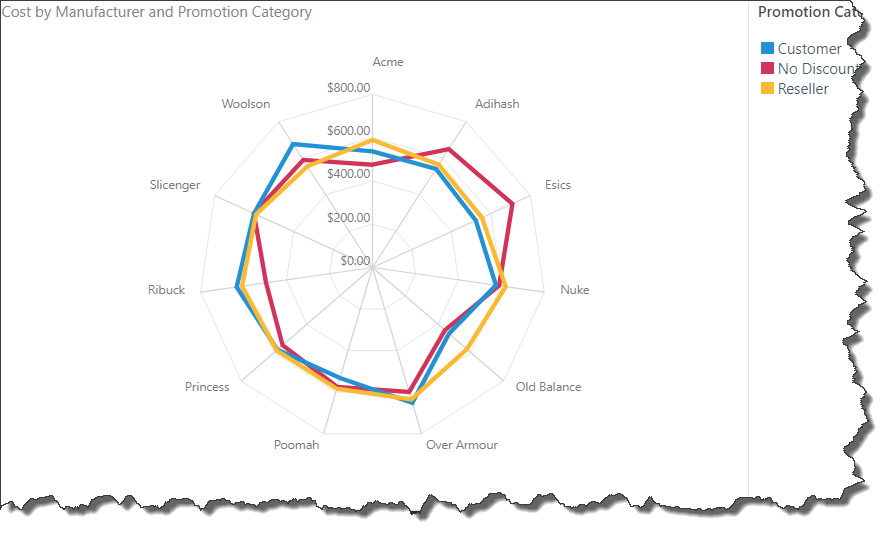To build a radar chart, you must add a measure to the Values zone and a hierarchy to Categories.

The hierarchy in Categories determines the number of axes in the chart, with each axis representing a category (green arrow). The measure drives the values in the chart (purple highlight below).

At this point, the chart is showing cost for each manufacturer; in order to plot another series on the chart, another hierarchy must be added to the Color drop zone.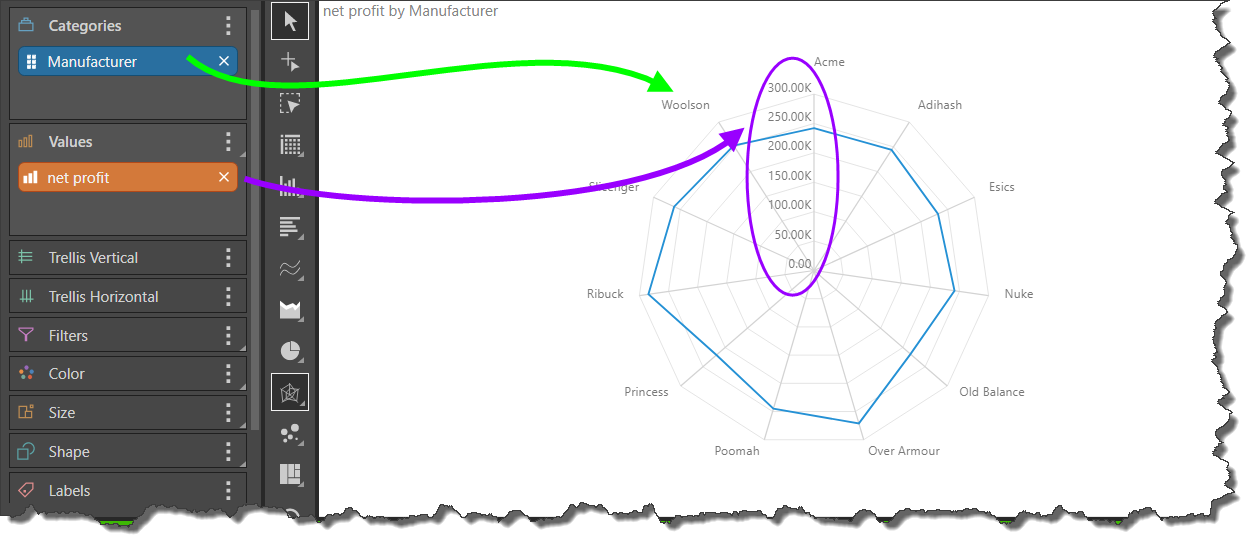To plot another series across the axes, add a member element to the Color zone. In this example, the Reseller element from the Promotion Category hierarchy was added.

The chart now shows cost for the Reseller promotion by each manufacturer.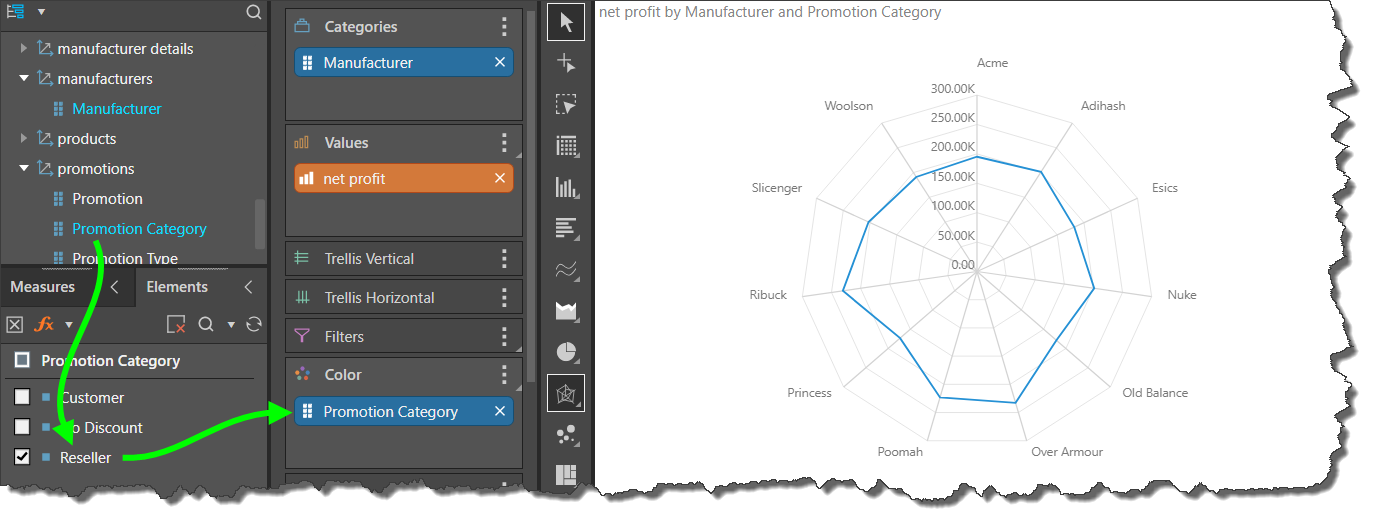To plot multiple series across the axes, add a hierarchy or multiple member elements to the Color zone. The hierarchy added to Color drives the number of series (lines) in the chart and their color (yellow arrows below).

Here, the entire Promotion Category hierarchy was added, plotting 3 series across the axes. So the radar chart below displays Cost for Promotion Category for each Manufacturer; we see that the cost for the Customer promotion for Old Balance was about \$500,000, while for Esics it was about \$700,000.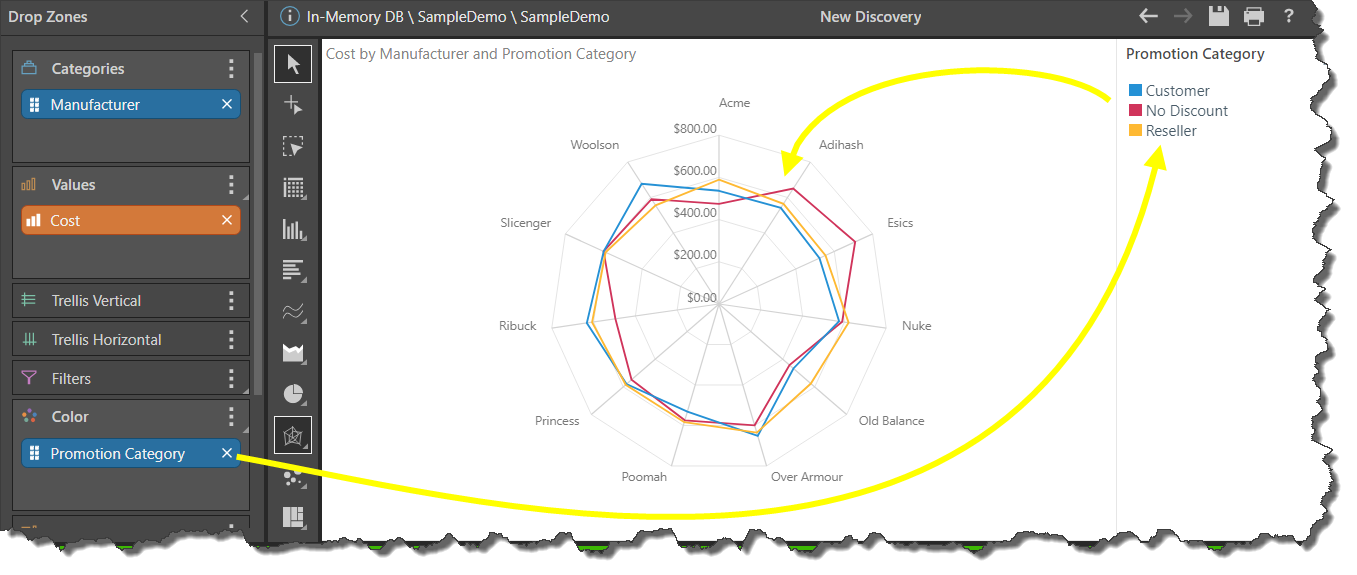The radar line chart plots the series' data points on each axis, and connects them with a straight line.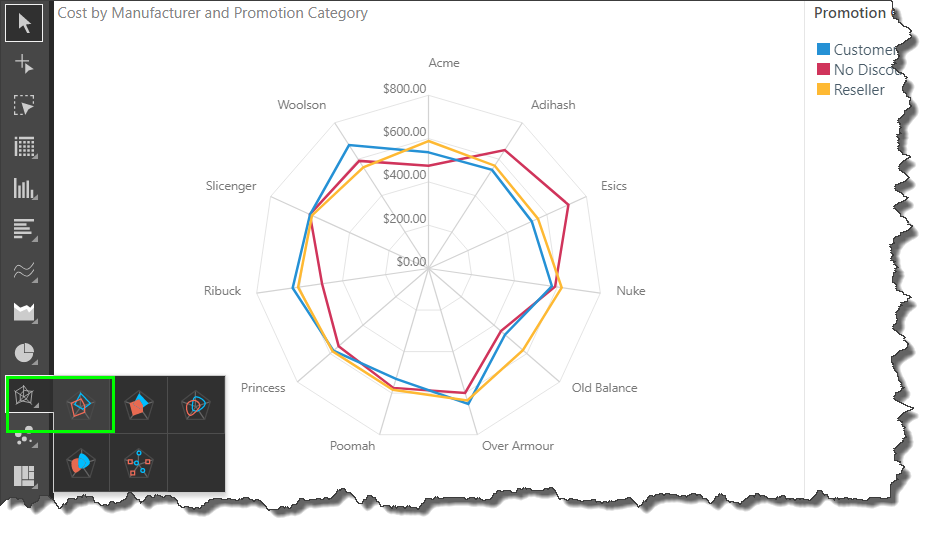The radar area chart plots the data points of each series on each axis, and connects the points to draw a shaded polygon for each series. Each polygon is overlaid one over the other, with reduced opacity enabling you to see the variations.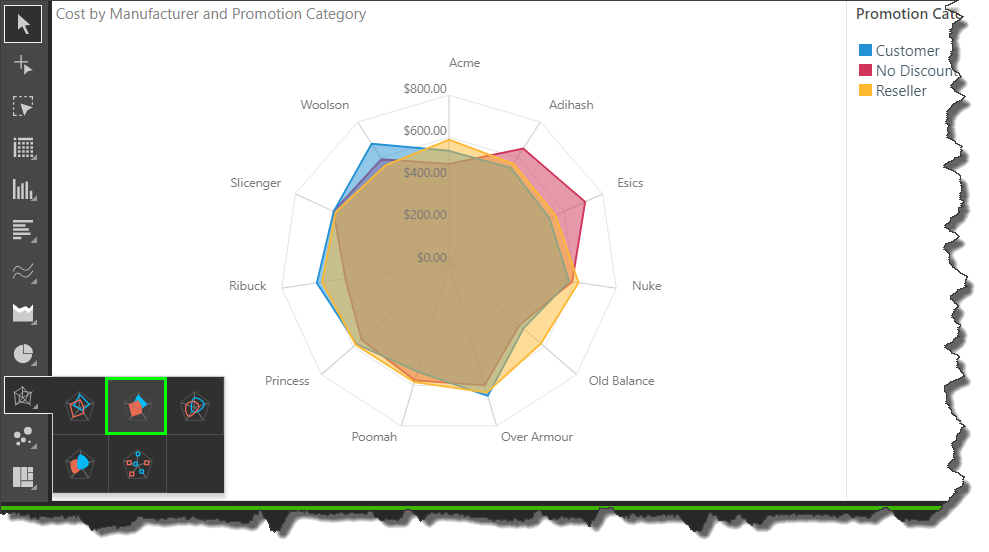Radar smooth line charts plot the data points for each series and connect them with a curved line. The difference between the radar line chart and the radar smooth line chart is cosmetic, although if there are many axes and observations, it may be easier to differentiate the data points when a straight line is used.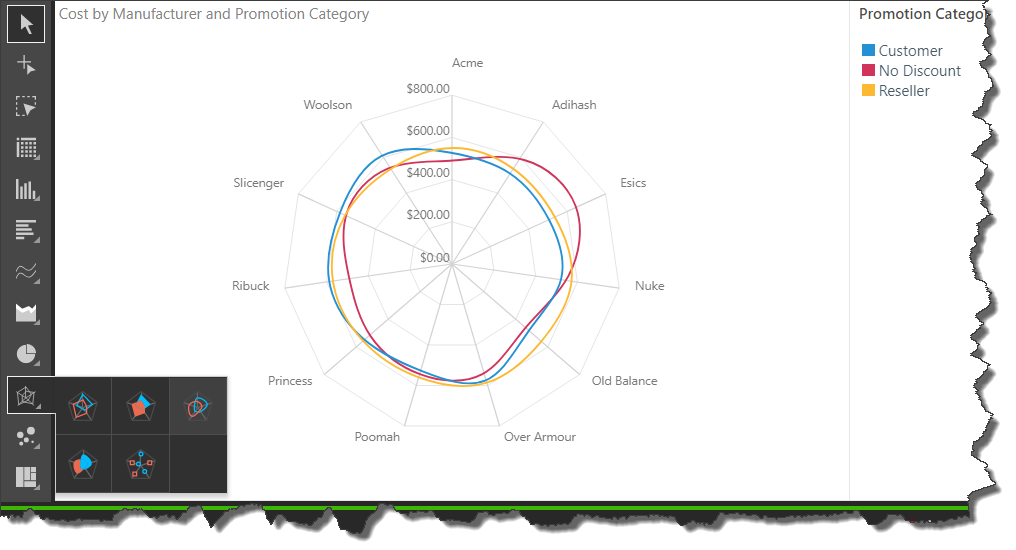### Radar Area Smooth Line Chart

Radar area smooth line charts plot the data points of each observation on each axis, and connect the points with a curved line, filling in the area to draw a curved shape for each observation.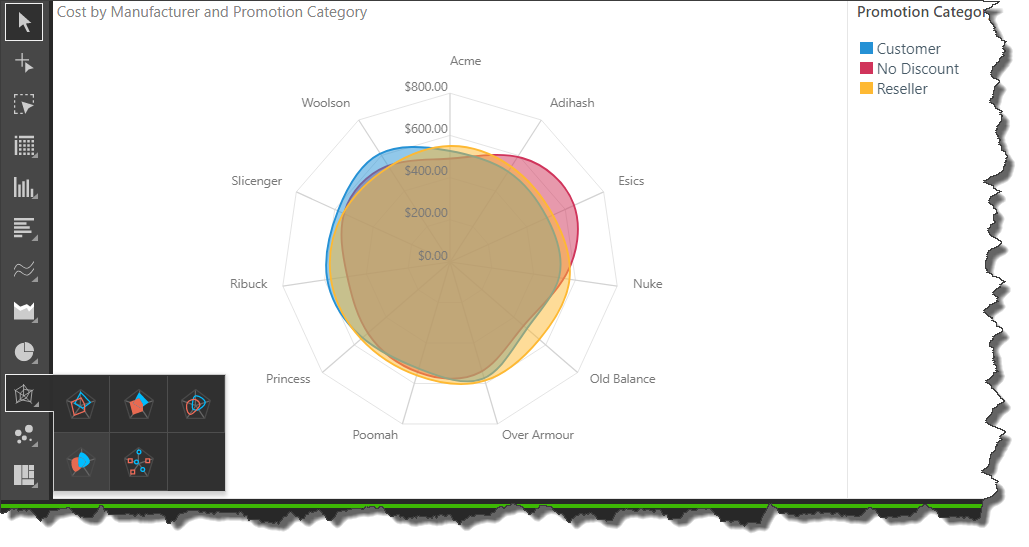The radar point chart plots the data points for each observation along each axis, but does not connect the data points.## Examples

In the example below, a radar line chart is used to plot the cost for Promotion Categories for each Manufacturer. The Product Category hierarchy was then added to Trellis Horizontal, producing a separate radar chart for each product category. In this way, the user can easily compare each series across different categories in a given hierarchy.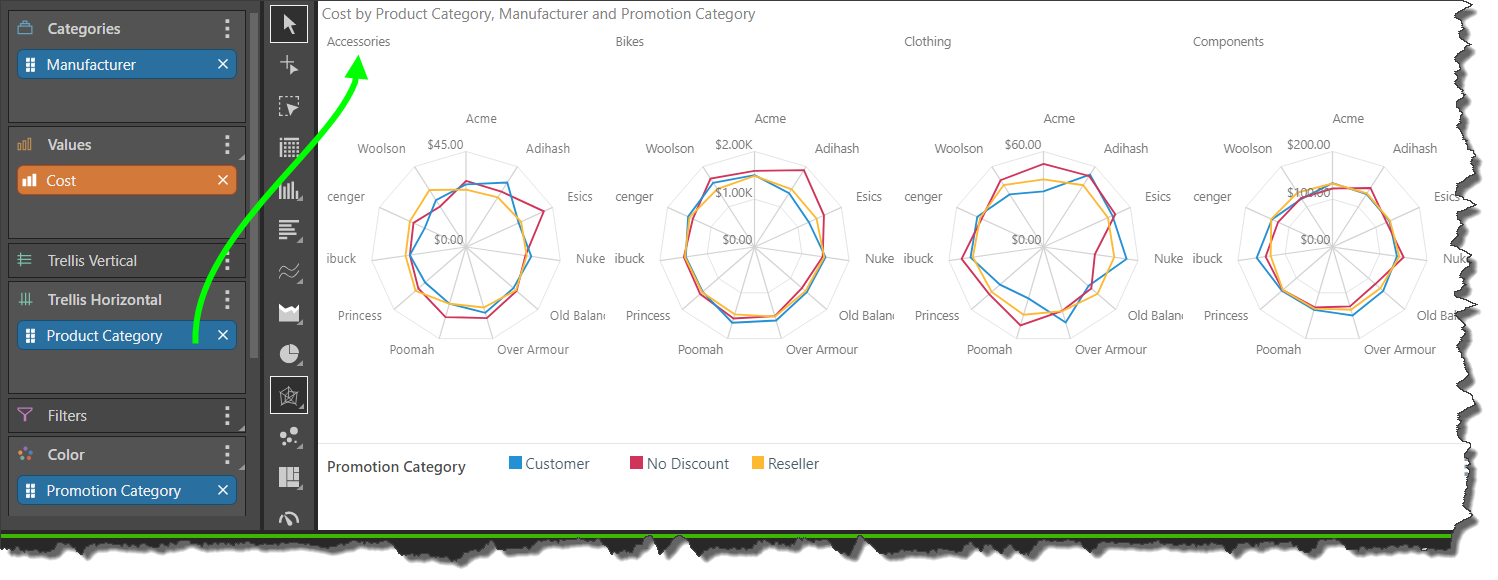In cases where you need to plot many series of values, the radar chart can quickly become illegible. In these instances, it's best to avoid 'overplotting' by utilizing the trellis function; rather than adding the second hierarchy to Color, add it to Trellis to produce multiple charts, with each chart plotting a single series.

In the example below, Net Profit by Month is shows for each Manufacturer. Rather than overlaying 12 months in a single chart, the chart was trellised by month, so that each chart represents a single month.